# Equivalence Rule in DBMS

As we saw above, any two relational expressions are said to be equivalent, if both the expression generate same set of records. When two expressions are equivalent we can use them interchangeably. i.e.; we can use either of the expression whichever gives better performance.

We can have different equivalent expression for different types of operations. Equivalence Rule defines how to write equivalence expression for each of the operators. Let us see them below.

## Selection Operations

When we have multiple selection operation looping one inside other on the table, then we can write them in any order. That is,

σθ1 (σθ2 (T)) = σθ2 (σθ1 (T)), where T is the table and θ is filter condition.

This implies that in a selection operation order of θ1 and θ2 does not affect the result. It can be used in any order. That is conditions of selection operation are commutative in nature.

For example, retrieve the students of age 18 who are studying in class DESIGN_01.

```SELECT * FROM (SELECT *
FROM STUDENT WHERE AGE = 18) t
WHERE CLASS_ID = ‘DESIGN_01’;```

or

```SELECT * FROM (SELECT *
FROM STUDENT WHERE CLASS_ID = ‘DESIGN_01’) t
WHERE AGE =18;```

(Both these queries are same and yield same result.)

Actually, relational expressions are written in this form as a part of equivalence relation. Above query is written for better understanding. Going forward, let us try to understand the equivalence rule in terms of relational expression. Relational expression for above query can be written as below:

σ AGE = 18 (σCLASS_ID = ‘DESIGN_01’ (STUDENT)) = σCLASS_ID = ‘DESIGN_01’ (σ AGE = 18 (STUDENT))

Let us see this with the help of data. Below tables show the select operation by altering the filtering condition. Though the intermediate tables will have different records, the final result is same.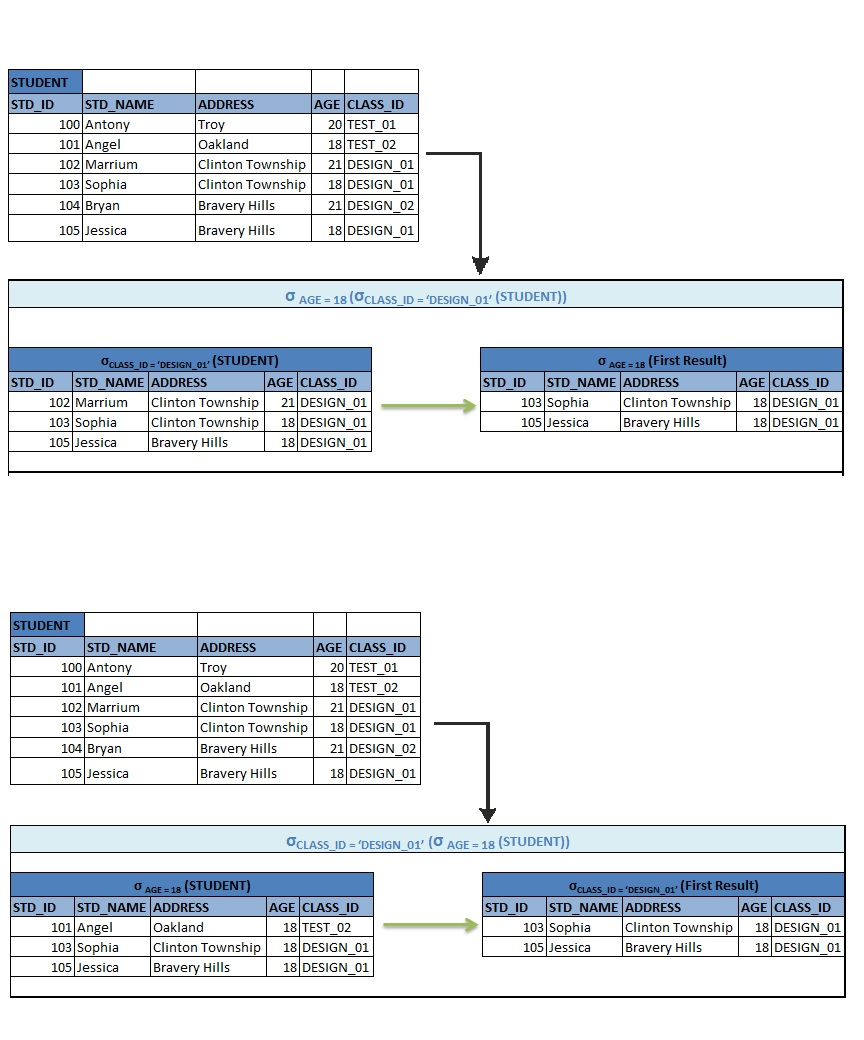## Conjunctive Selection

When we have selection operation with multiple filter conditions, then we can split them into sequence of selection operation. That is,

σ θ1 AND θ2(T)) = σ θ1(σθ2(T))

For example, retrieve the students of age 18 and who are studying in class DESIGN_01.

σ AGE = 18 AND CLASS_ID = ‘DESIGN_01’ (STUDENT) = σ AGE = 18 (σCLASS_ID = ‘DESIGN_01’ (STUDENT))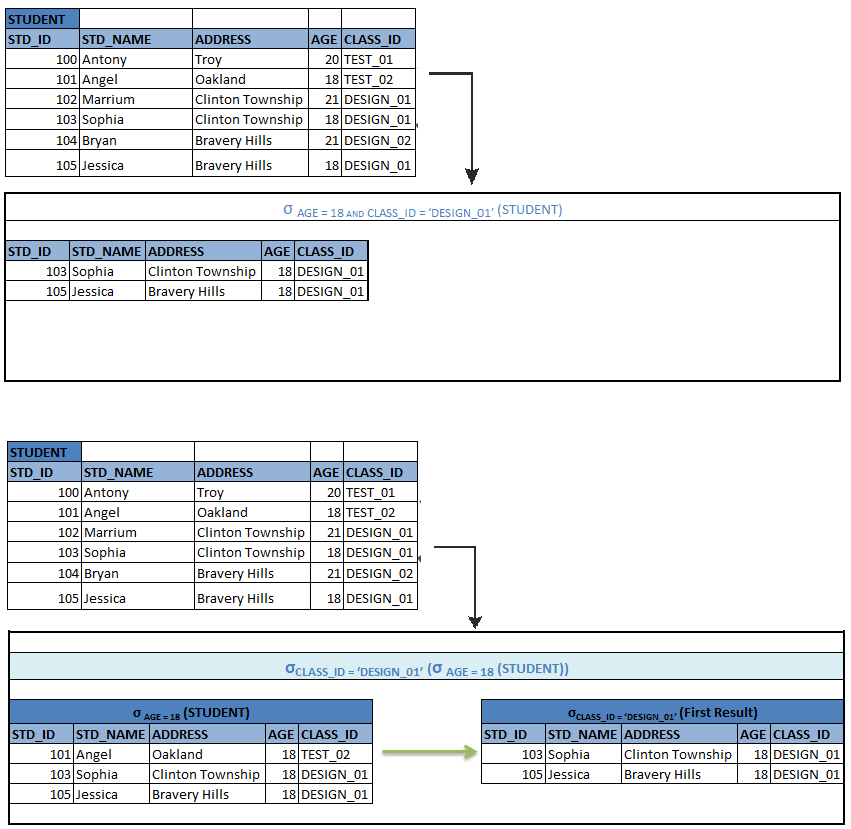## Sequence of projection operation

When there is a sequence of projection operation, only the last projection is required and rest of the projections can be ignored. In terms of relational expression, it can be written as below:

```∏Col_list1 (∏Col_list2 (… (∏Col_listN (T))….)) = ∏Col_list1 (T)

∏ STD_ID, STD_NAME (∏STD_ID, STD_NAME, AGE, ADDRESS (∏STD_ID, STD_NAME, AGE, ADDRESS, CLASS_ID, SKILLS

(STUDENT))) = ∏STD_ID, STD_NAME (STUDENT)```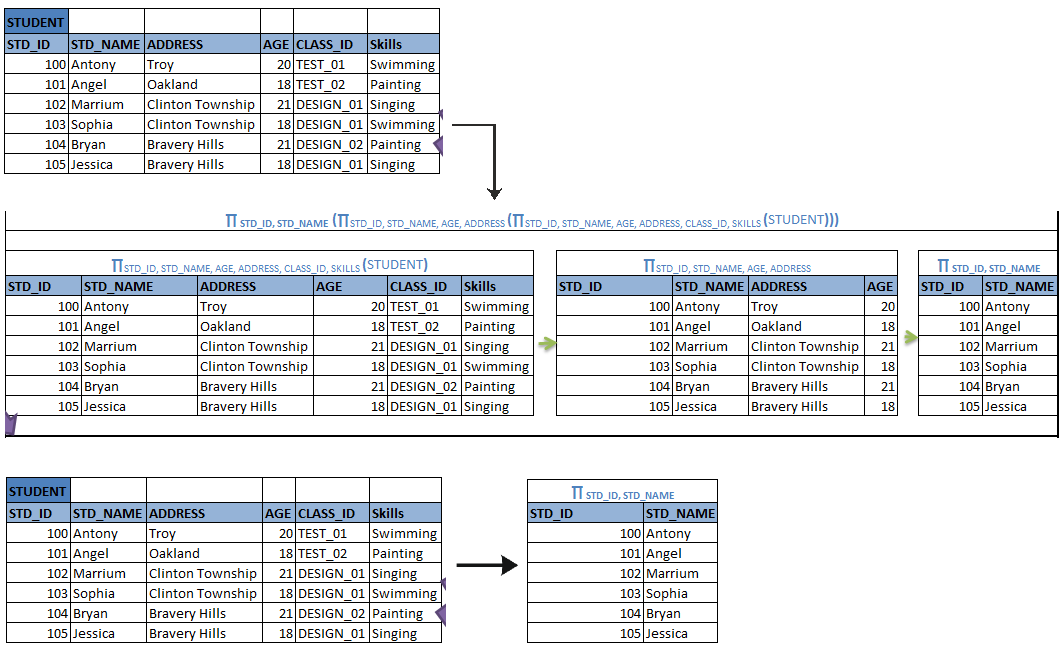That means final set of columns which we are going to select is only required rather than selecting all set of different columns throughout the query.

## Selection with Cartesian product and Joins

When we have Cartesian product on two tables and a selection condition on the result, then we can replace it with natural join with filter condition.

```σ θ1 (T X S) = T ∞ θ1S

σ e.DEPT_ID = d.DEPT_ID (EMP X DEPT) = EMP ∞ e.DEPT_ID = d.DEPT_ID DEPT```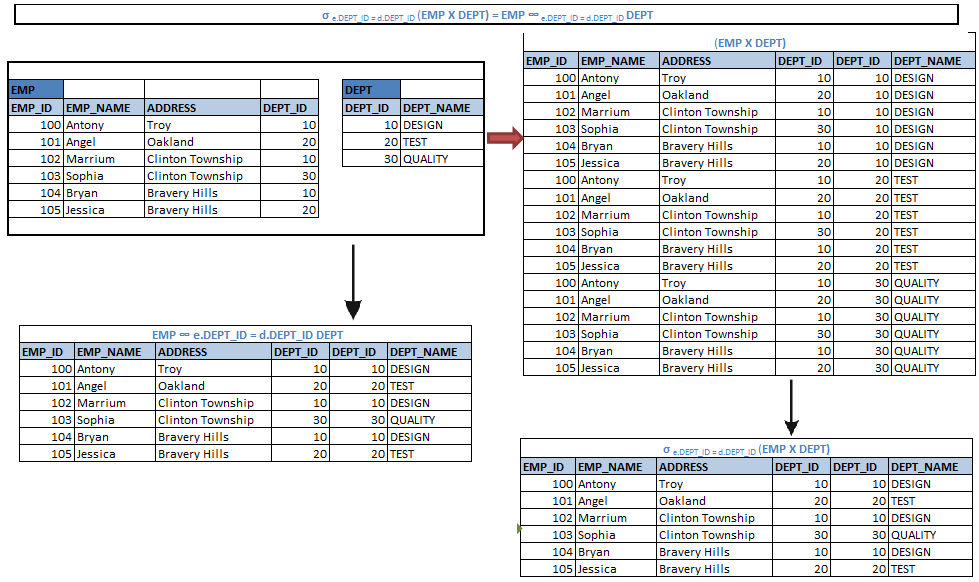i.e.; the Cartesian product on EMP and DEPT will combine the records of both the tables irrespective of the any joins. When a selection operation with join condition is applied on it, it selects only those records which have same DEPT_ID. The same can be done with natural join where it selects only the matching records from both the tables based on DEPT_ID.

Similarly, when we have natural join on two tables with one condition and a selection operation on it with another condition, then we can combine the conditions into natural join.

```σ θ1 (T ∞ θ2S) = T ∞ θ1 AND θ2S

σ AGE = 23 (EMP∞ e.DEPT_ID = d.DEPT_ID DEPT) = EMP ∞ AGE =23 AND e.DEPT_ID = d.DEPT_ID DEPT```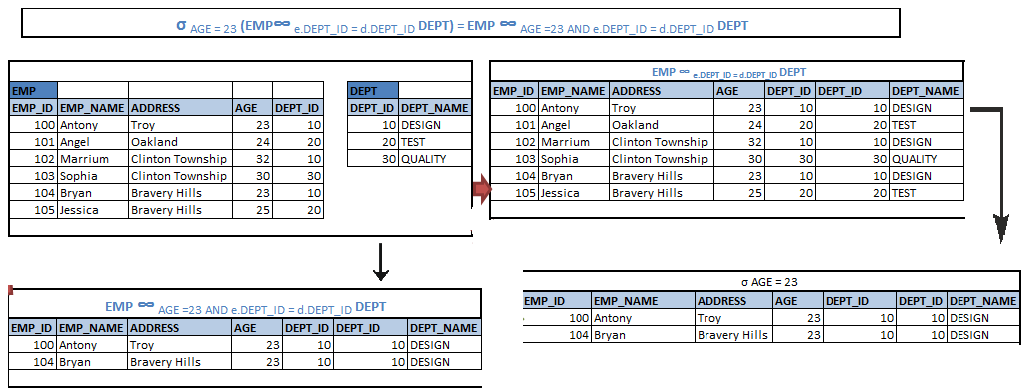i.e.; When a natural join on EMP and DEPT is performed based on DEPT_ID, and then the result is filtered for AGE = 23 will yield a same result as performing natural join with condition on DEPT_ID and AGE = 23.

## Natural Join with θ Join

When we have natural join on two tables with θ join, then the order of the tables does not have any significance. That means we can have tables in any order implying that they are commutative.

```T ∞ θ2 S = S ∞ θ2 T

EMP ∞ e.DEPT_ID = d.DEPT_ID DEPT = DEPT ∞ e.DEPT_ID = d.DEPT_ID EMP```

This is straight forward

### Associative Natural Joins

This is the enhancement of above rule. It states that when natural joins are performed on three or more tables, then it can be performed in any combination of two tables. That means natural joins are associative.

```(R ∞ S)  ∞ T = R ∞ (S ∞ T)

(EMP ∞ DEPT)  ∞ PROJECT = EMP ∞ (DEPT ∞ PROJECT)```

Similarly, theta joins are also associative.

```(R ∞θ1 S)  ∞ Θ2 AND θ3 T = R ∞ θ1 AND θ3 (S ∞ θ2 T)
(EMP ∞ e.DEPT_ID = d.DEPT_ID DEPT)  ∞d.PROJ_ID = p.PROJ_ID AND e.PROJ_ID = p.PROJ_ID PROJECT = EMP ∞ e.DEPT_ID = d.DEPT_ID AND e.PROJ_ID = p.PROJ_ID (DEPT ∞d.PROJ_ID = p.PROJ_ID PROJECT)```

## Distributive Selection Operation

Selection operation with theta join (natural join) are distributive as below:

• When all the columns of select conditions have only one table, then we can re-write selection with theta join as below:
```σ θ1 (T ∞ θ2S) = (σ θ1 T) ∞ θ2S
σ AGE = 18 (EMP ∞ e.DEPT_ID = d.DEPT_ID DEPT) = (σ AGE = 23 EMP) ∞ e.DEPT_ID = d.DEPT_ID DEPT```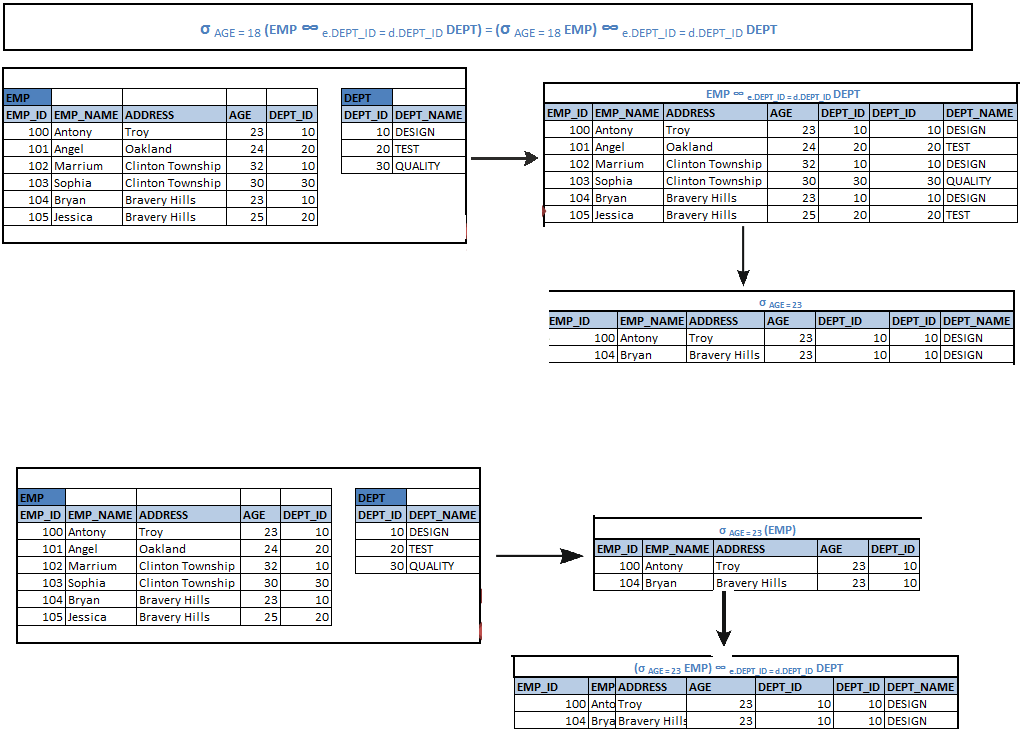Joining the tables with less number of records is always efficient. Performing SELECT operation first will reduce number of records to be joined in a relation, hence increasing the performance. This is the inference we get from above rule.

• When the condition θ1 has only the columns of T and θ2 has only the columns of S and θ has columns from both the tables, then we can have equivalence rule as below.
```σ θ1 AND θ2 (T ∞ θ S) = (σ θ1 T) ∞ θ (σ θ2S)
σ AGE=23 AND DEPT_NAME = ‘DESIGN’ (EMP ∞ e.DEPT_ID = d.DEPT_ID DEPT) = (σ AGE=23 EMP) ∞ e.DEPT_ID = d.DEPT_ID (σ DEPT_NAME = ‘DESIGN’ DEPT)```

## Distributive Projection Over Theta Join

Like selection operation, projection operation is also distributive.
Let CT and CS be the columns of T and S respectively. Then selecting the columns of T and S after having theta join on them is equivalent to selecting the columns of individual tables and then having join on them.

```∏ CT U CS (T ∞ θ S) = ∏ CT (T) ∞ θ∏ CS (S)
∏ EMP_ID, EMP_NAME, DEPT_ID, DEPT_NAME (EMP∞ DEPT_ID DEPT) = ∏ EMP_ID, EMP_NAME (EMP) ∞ DEPT_ID ∏ DEPT_ID, DEPT_NAME (DEPT)```
• Let CT and CS be the columns of T and S respectively and are selected in the final result. Let CT1 and CS2 be the columns of T and S respectively and are used in the theta join, but are not in CT and CS. Then selecting the columns of T and S after having theta join on them is equivalent to selecting the columns of individual tables and then having join on them.
```∏ CT U CS (T ∞ θ S) = ∏ CT U CS ((∏ CT U CT1 T) ∞ θ (∏ CS U CS1 T))
∏ EMP_ID, EMP_NAME, DEPT_ID, DEPT_NAME (EMP ∞ θ DEPT) = ∏ EMP_ID, EMP_NAME, DEPT_ID, DEPT_NAME ((∏EMP_ID, EMP_NAME, SALARY EMP) ∞ θ (∏DEPT_ID, DEPT_NAME, NUM_PROJ DEPT))
SELECT EMP_ID, EMP_NAME, DEPT_ID, DEPT_NAME
FROM ((SELECT * FROM EMP e, DEPT d
WHERE e.DEPT_ID = d.DEPT_ID AND SALARY >=30000 AND NUM_PROJ>10));```

Is equivalent to:

```SELECT EMP_ID, EMP_NAME, DEPT_ID, DEPT_NAME
FROM (
(SELECT EMP_ID, EMP_NAME, SALARY, DEPT_ID FROM EMP) e,
(SELECT DEPT_ID, DEPT_NAME, NUM_PROJ FROM DEPT) d
WHERE e.DEPT_ID = d.DEPT_ID AND SALARY >=30000 AND NUM_PROJ>10) ;```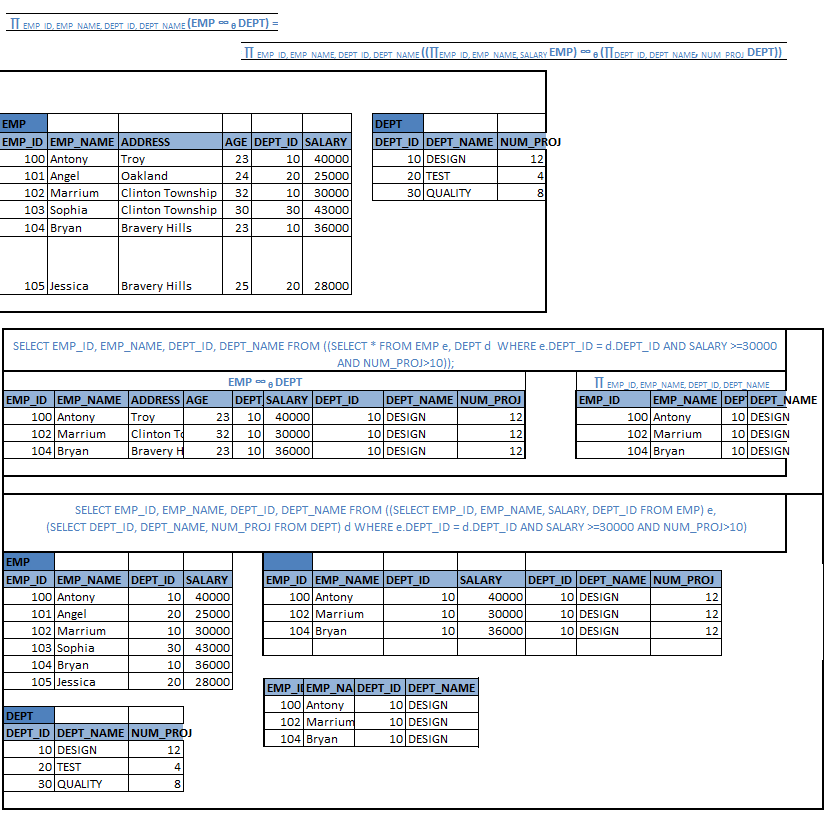## Commutative Set Operation

This rule states that tables used in set operators like UNION and INTERSECTION can be used interchangeably. i.e.;

T U S = S U T
T ∩S = S ∩T

Eg :

DESIGN_EMP U TEST_EMP = TEST_EMP U DESIGN_EMP
DESIGN_EMP ∩ TEST_EMP = TEST_EMP ∩ DESIGN_EMP

## Associative Set Operation

This rule states that when there is a sequence of UNION or INTERSETION operation on more than two tables, and then it can be evaluated from beginning to end or from end to beginning by selecting the combination of two tables each time. i.e.;

R U (S U T) = (R U S) U T
R ∩ (S ∩ T) = (R ∩ S) ∩ T

Eg :

(DESIGN_EMP U TEST_EMP) U QC_EMP = DESIGN_EMP U (TEST_EMP U QC_EMP)
(DESIGN_EMP ∩ TEST_EMP) ∩ QC_EMP = DESIGN_EMP ∩ (TEST_EMP ∩ QC_EMP)

## Distributive Selection Operation over Set Operators

### This rule has two parts:

• First rule says that, selecting the records after applying Set operator is same as applying the Set operator on individual selection operation.

σ θ (T U S) = σ θ (T) U σ θ (S)
σ θ (T ∩ S) = σ θ (T) ∩ σ θ (S)
σ θ (T – S) = σ θ (T) – σ θ (S)

Eg :

σ θ (DESIGN_EMP U TEST_EMP) = σ θ (DESIGN_EMP) U σ θ (TEST_EMP)
σ θ (DESIGN_EMP ∩ TEST_EMP) = σ θ (DESIGN_EMP) ∩ σ θ (TEST_EMP)
σ θ (DESIGN_EMP – TEST_EMP) = σ θ (DESIGN_EMP) – σ θ (TEST_EMP)

• Second rule states that applying selection condition on first table after applying the set operator is same as applying selection condition on first table first and then applying the set operator.

σ θ (T U S) = σ θ (T) U S
σ θ (T ∩ S) = σ θ (T) ∩ S
σ θ (T – S) = σ θ (T) – S

Or

σ θ (DESIGN_EMP U TEST_EMP) = σ θ (DESIGN_EMP) U TEST_EMP
σ θ (DESIGN_EMP ∩ TEST_EMP) = σ θ (DESIGN_EMP) ∩ TEST_EMP
σ θ (DESIGN_EMP – TEST_EMP) = σ θ (DESIGN_EMP) – TEST_EMP

## Distributive Projection over Union operator

This rule says projecting the columns after applying UNION operator is same as projecting the columns of individual tables and then applying UNION operator.

∏ CT U CS (T U S) = ∏ CT (T) U ∏ CS (S)

Eg :

∏ EMP_NAME (DESIGN_EMP U TEST_EMP) = ∏ EMP_NAME (DESIGN_EMP) U ∏ EMP_NAME (TEST_EMP)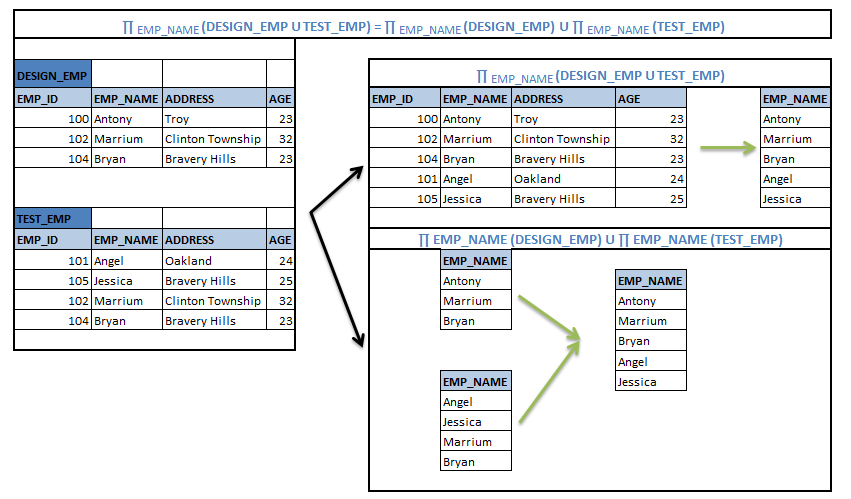When there is complex query, the processor will analyze and see which of above rule can be applied on it, so that processing becomes efficient and easier. Some of the key points to be noted while evaluating the query are

• Executing the queries with less number of records is always efficient. Hence perform selection operation as early as possible, so that it reduces the number of records in the query.
For example, retrieve student names, who are studying in class DESIGN_01. This can be done in two ways. First, retrieve all the student names and class from STUDENT table, and then apply the filter to select only DESIGN_01 students. Suppose we have 100 students in the student table and only 10 are in DESIGN_01 class. Then below query will retrieve student name and CLASS_ID for all the 100 students first, then it will apply filter to select the 10 design students. The cost of query is hence more in this case. In addition, it needs more space to store temporary result of STD_NAME and CLASS_ID for 100 students.

∏STD_NAME (σ CLASS_ID = ‘DESIGN_01’ (∏STD_NAME, CLASS_ID (STUDENT)))

Second method is to filter students who are studying in class DESIGN_01. Then select student names from this list of records. Here, it filters DESIGN_01 students first. Hence it selects only 10 student records. It then selects only required column – STD_NAME. This is more efficient method, as it reduces the number of records at first step itself.

∏STD_NAME ( σ CLASS_ID = ‘DESIGN_01’ (STUDENT))

• When there is multiple tables involved in a query with different joins, then it is better to split the query into different tokens and then execute the query in a sequence. We can apply above 12 rules in this situation.
Suppose we have to retrieve the details of employees who work at DESIGN department and are of age 23. The query can be written as below:

σ AGE=23 AND DEPT_NAME = ‘DESIGN’ (EMP ∞ e.DEPT_ID = d.DEPT_ID DEPT)

But selecting the required records at the end will have large number of data till the end. Hence it is better to filter number of records in each table first, and then applying the join is better. Hence first task is to filter EMP records, and then Filter DEPT records, then join them. These actions will be performed in a sequence.

(σ AGE=23 EMP) ∞ e.DEPT_ID = d.DEPT_ID (σ DEPT_NAME = ‘DESIGN’ DEPT)

• When there is only few set of columns needs to be displayed in the result, then push the projection operation as early as possible. This is similar to pushing the selection operation. Having selection and projection early in the query reduces the number of unnecessary records and attributes in the temporary tables. We can use any of 12 equivalence rule to get this task done.

∏ EMP_ID, EMP_NAME, DEPT_ID, DEPT_NAME (EMP∞ DEPT_ID DEPT) = ∏ EMP_ID, EMP_NAME (EMP) ∞ DEPT_ID ∏ DEPT_ID, DEPT_NAME (DEPT)

• While joining more than two tables, we can use join associative rule, so that we can join smaller table first, hence the size of the temporary table is small.
Suppose, we have EMP, DEPT and PROJ tables with records 10000, 20, 50 records respectively. Suppose we have to retrieve EMP_NAME, DEPT_NAME and PROJ_NAME for each employee. Then the query would be:

EMP ∞DEPT_ID DEPT ∞ PROJ_ID PROJ

Here we can start evaluating from beginning to end or from end to beginning. But starting from beginning has very large table, EMP. Hence the intermediary temporary table created will need large space to hold the records of joining. Hence it is better to evaluate from end to beginning. This uses the join associative rule to evaluate.

(EMP ∞DEPT_ID DEPT) ∞ PROJ_ID PROJ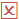EMP ∞DEPT_ID (DEPT ∞ PROJ_ID PROJ)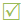Translate »